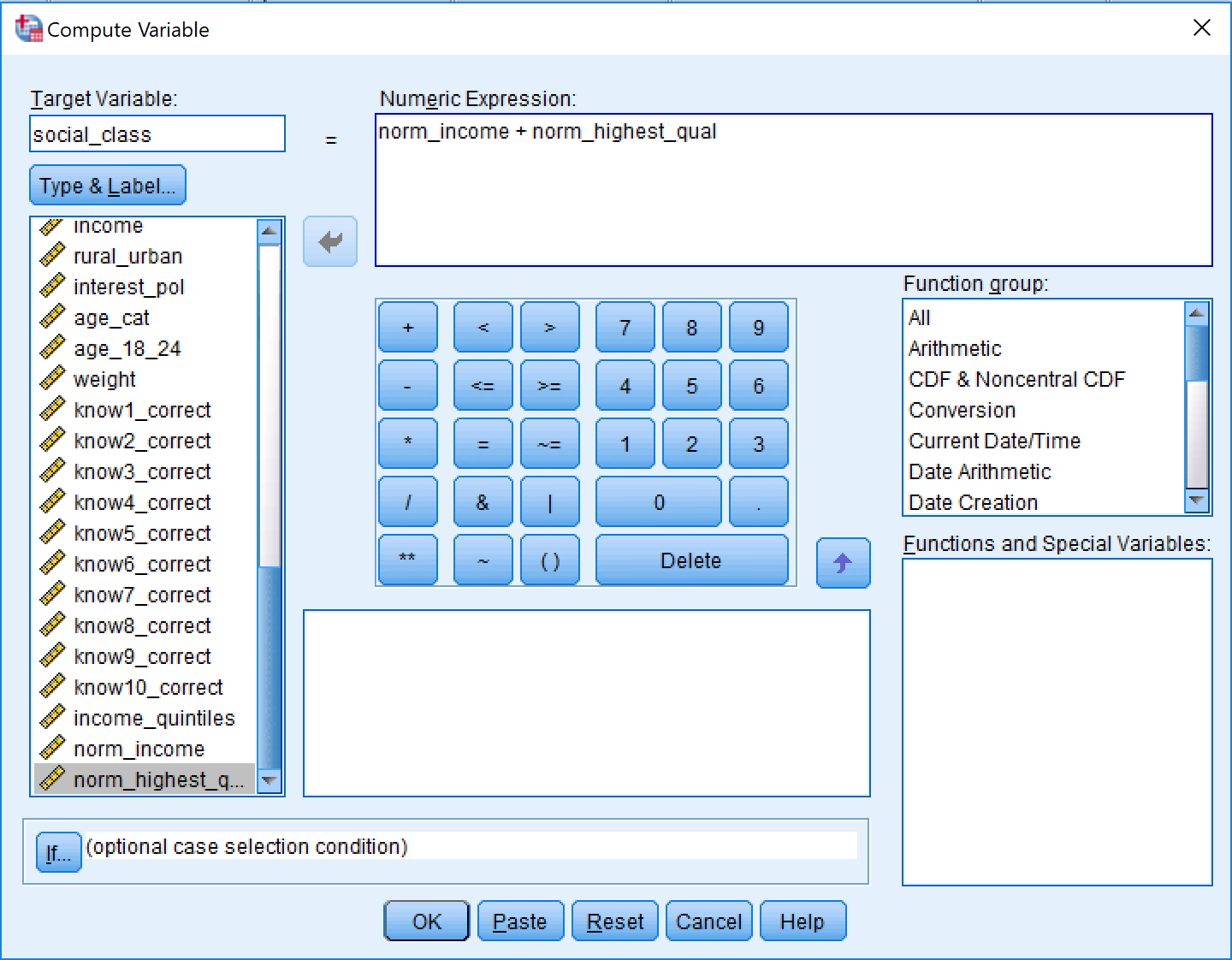# Summary

If you are analysing a survey, chances are you have many different questions which test for the same underlying theoretical concept.

When you have this situation, often the best thing to do is to construct an index.

There are generally three stages to constructing an index:

1. Normalization getting all variables onto the same scale (generally done with normalization);
2. Checking internal consistency checking that scales are all moving together in a reliable fashion (generally done by measuring the Cronbach Alpha and making sure it is > 0.6);
3. Summing the variables adding together all the variables into a new variable: the index.

Note that you can skip step 1 if your variables are all measured on the same scale (e.g. they are five answers to five point Likert scale).

# Step 1: Getting all variables into the same scale (normalization).

If our variables aren’t measured on the same scale, then the easiest thing to do is to normalize the variables.

1. Get the descriptive statistics for your variables: Analyse>Descriptive Statistics>Descriptives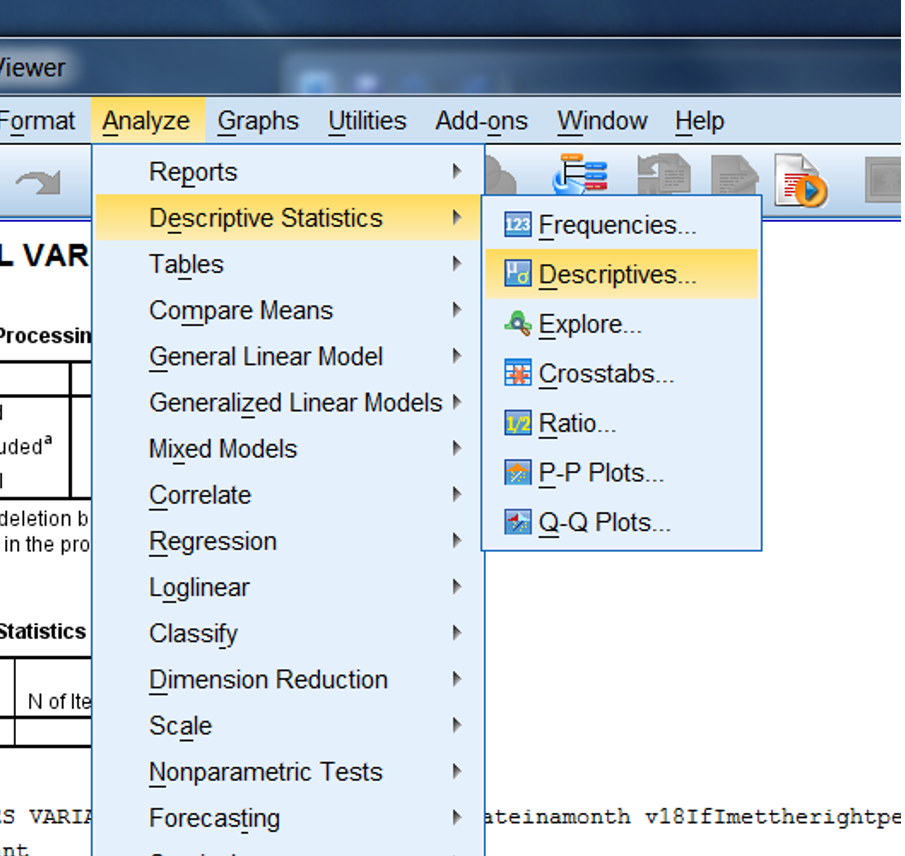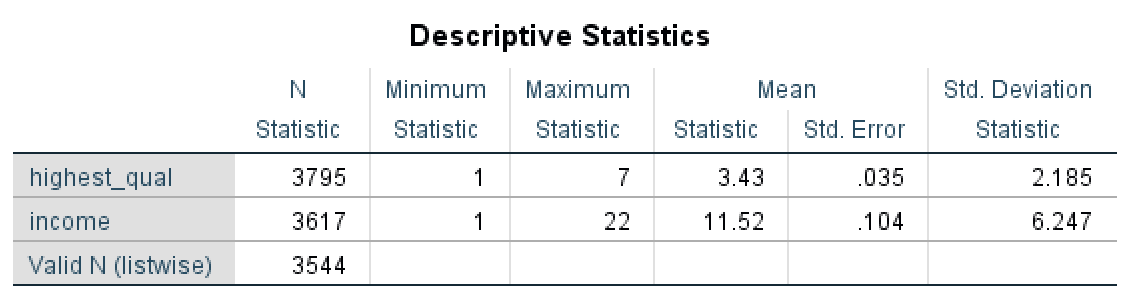1. Write down the mean and standard deviation for each variable.

2. Go to Transform>Compute Variable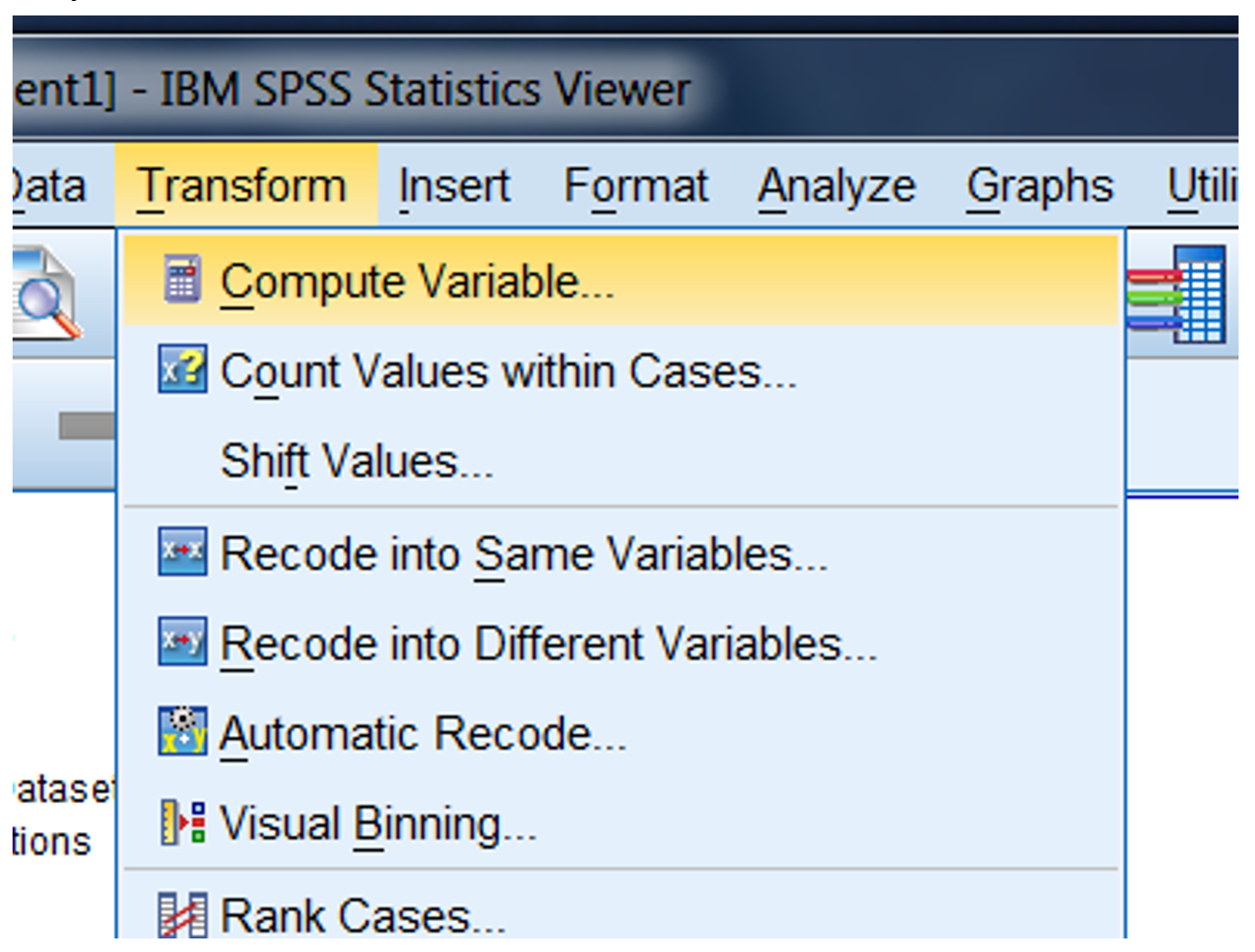1. In the dialogue box that comes up, type in a new target variable (in this case v16_normalized), and the normalizing equation:

$A^{normalised}_i = (A_i - A_{Mean})/A_{St Dev}$

Press OK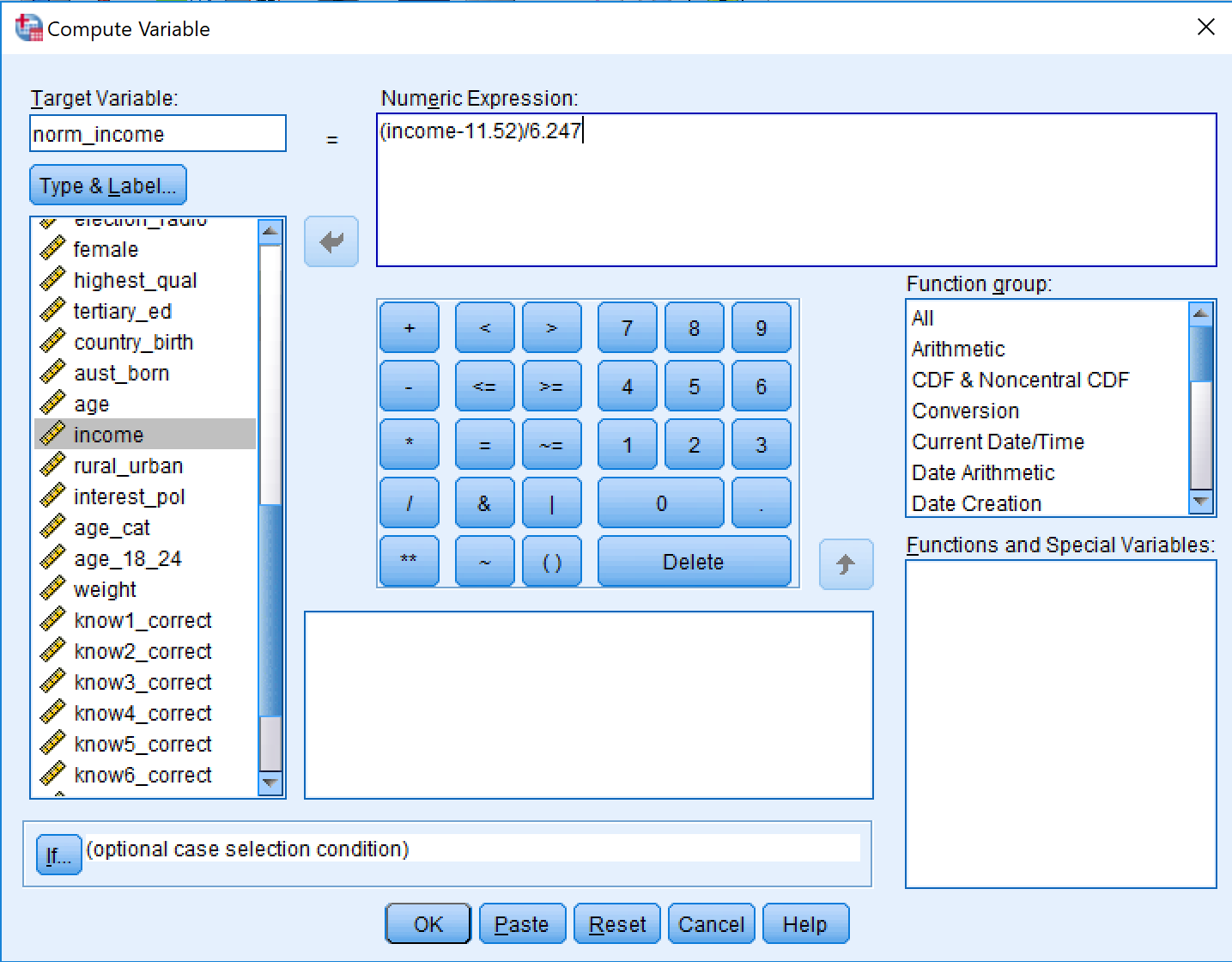A new variable will have been created. Take a look and make sure it looks like it has a mean of 0.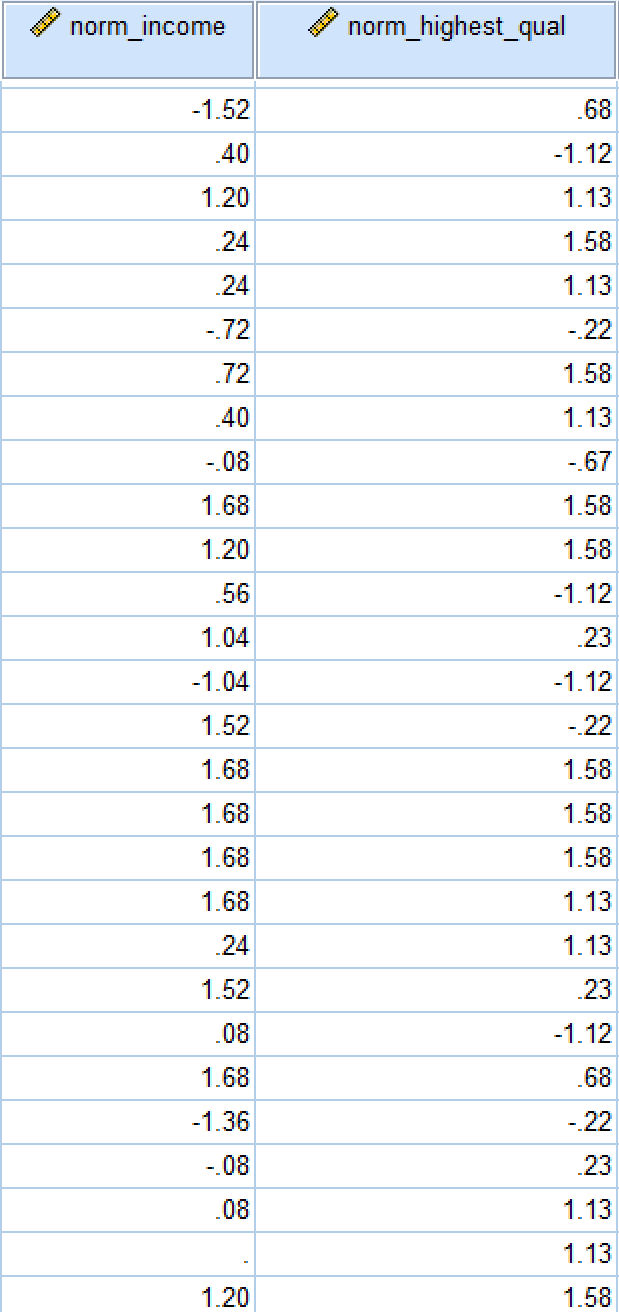1. Repeat this process of normalisation with your other variables.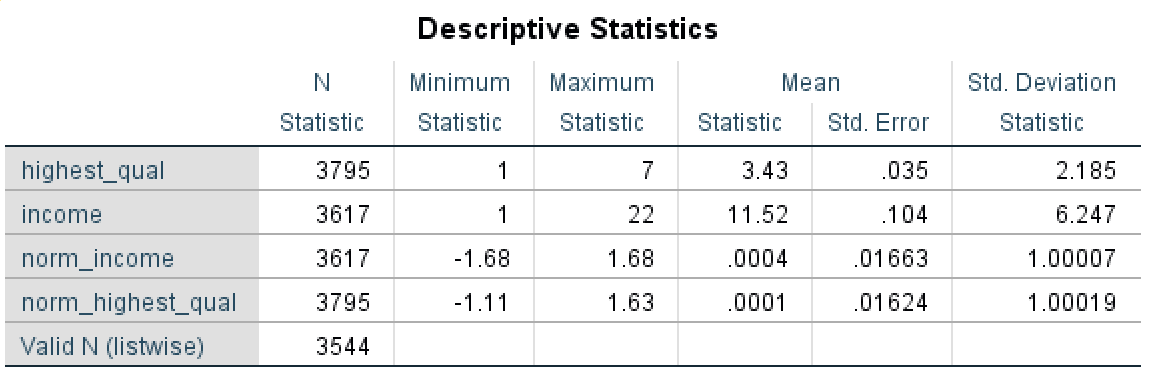# Step 2: Checking that the variables move together reliably (Checking the Cronbach Alpha)

1. Analyze > Scale > Reliability Analysis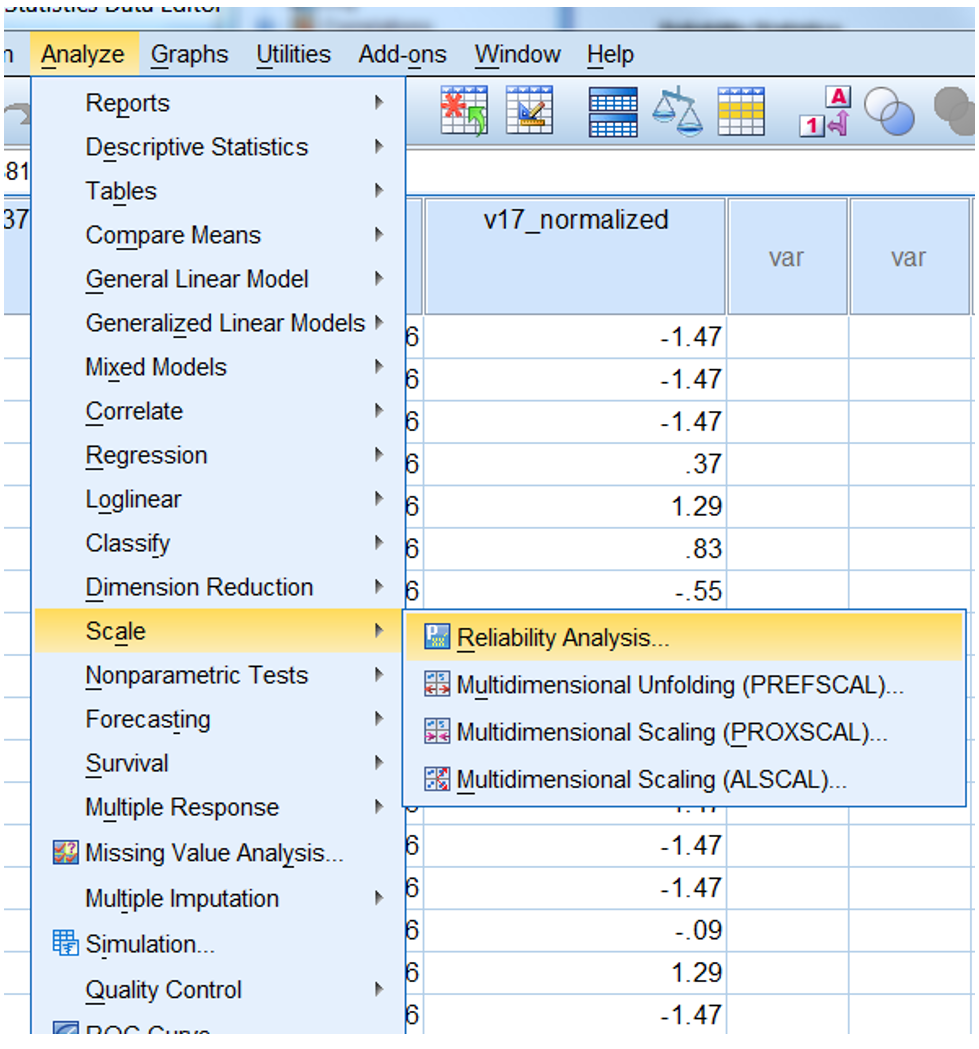1. Move the new normalized variables to the right side. Then click OK.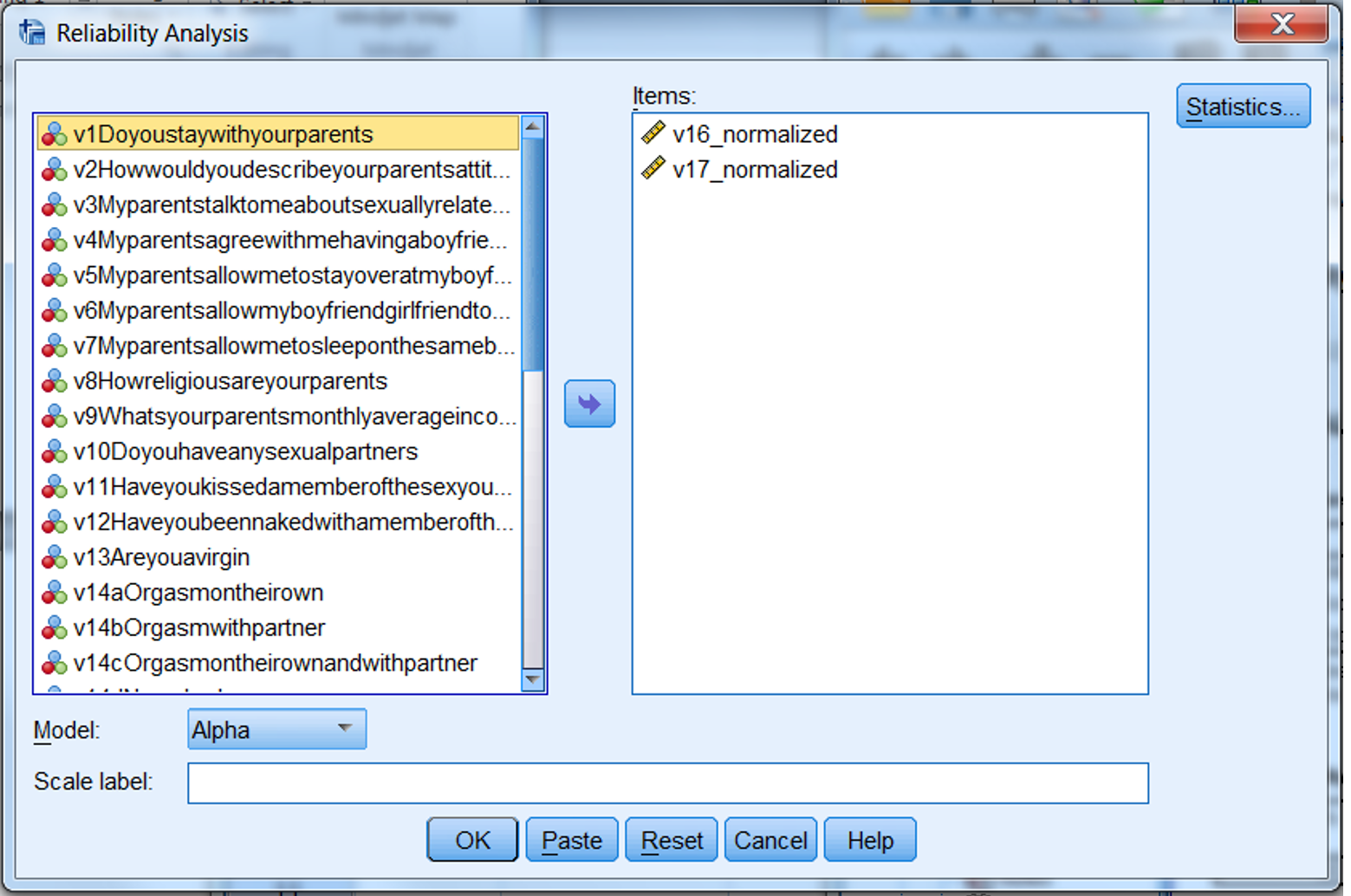1. Read the Cronbach’s Alpha from the output screen.

How to read this output? The normal cut off for most indexes is 0.6, though it is always better if it is higher than this.

You can see in our case, the reliability is a bit too low. We probably want to NOT construction an index, but instead treat these as two separate variables.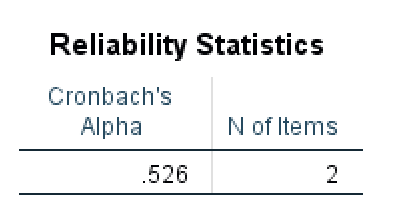1. If you are interested, at this stage you can check to see how much the normalization improved the Cronbach’s Alpha by running the same test on your original variables (in our case v16 and v17)

You can see that the Cronbach’s Alpha for our original variables was only 0.368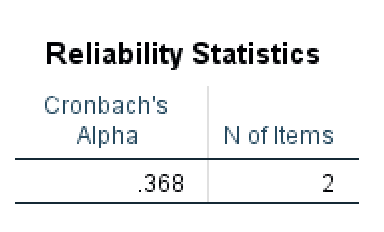# Step 3: Create the index

1. You now create the index by going back to Compute Variable: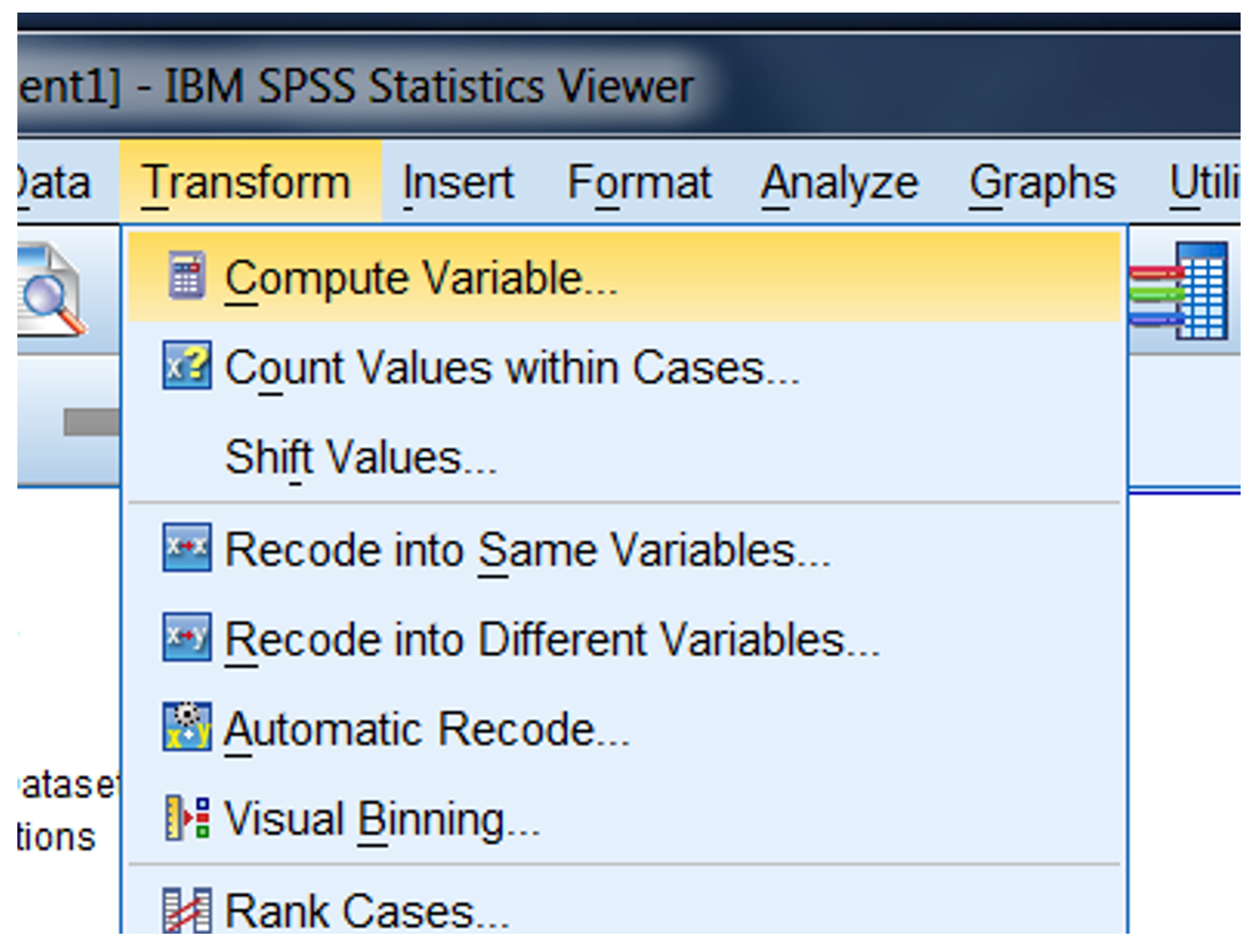1. Create a new variable called ‘index###’ by summing the normalized variables.